# Chem 130 Exam 1 No Answers - Chem 130: Exam one Practice...

• Test Prep
• 11
• 63% (8) 5 out of 8 people found this document helpful

This preview shows page 1 - 3 out of 11 pages.

The preview shows page 2 - 3 out of 11 pages.
Chem 130: Exam one Practice Test~~~~~~~~~~~~~~~~~~~~~~~~~~~~~~~~~~~~~~~~~~~~~~~~~~~~~~~~~~~~~~~~~~~~~~~1)Which statement about the scientific method is TRUE?A) The scientific method emphasizes reason as the way to understand the world.B) The scientific method emphasizes observation and reason as the way to understand theworld.C) The scientific method emphasizes observation and experimentation as the way to understandthe world.D) The scientific method emphasizes scientific laws as the way to understand the world.E) All of the above statements are false.
2)Who discovered the atomic theory?
3)What is the definition of a scientific theory?
4)There are exactly 2.54 centimeters in 1 inch. When using this conversion factor, how manysignificant figures are you limited to?
5)The correct number of significant figures in the number 865,000 is:A) 3B) 6C) 4D) ambiguousE) none of the above
##### We have textbook solutions for you!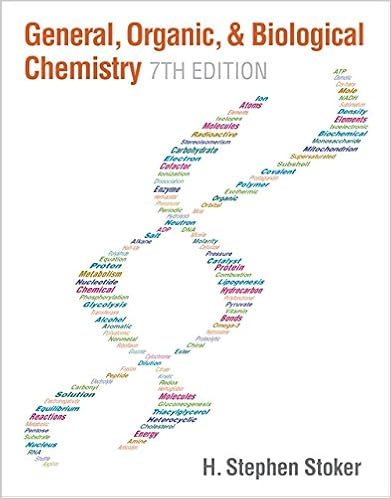The document you are viewing contains questions related to this textbook.
Chapter 2 / Exercise 2-87
General, Organic, & Biological Chemistry
StokerExpert Verified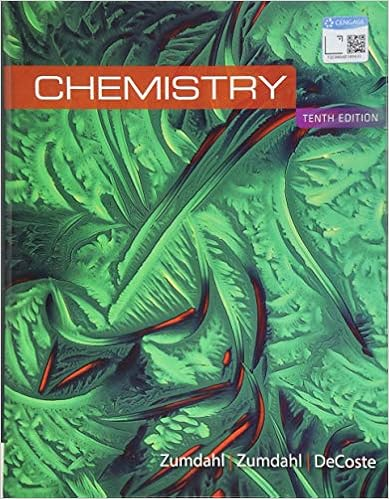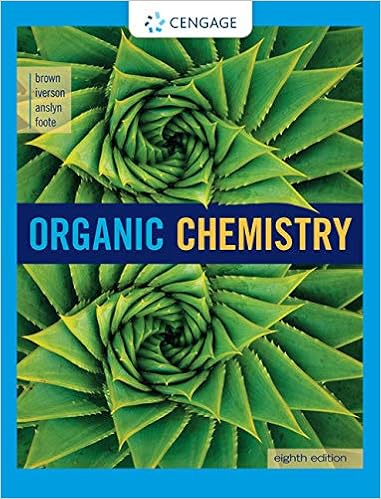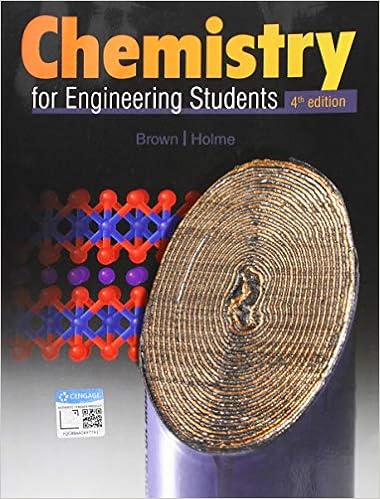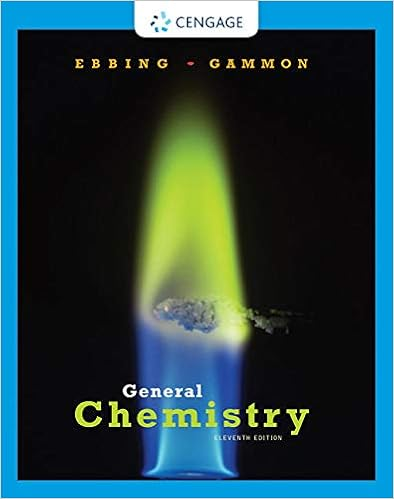Browse all Textbook Solutions
6)Determine the answer to the following equation with correct number of significant figures:(17.103 + 2.03) x 1.02521 = ________
7)The correct prefix for the multiplier 0.000001 is:
8)The correct multiplier for the prefix pico is:
9)Which of the following sets of units is not in the order of increasing size?A) μg < g < kgB) mL < dL < LC) ns < ms < sD) cm < μm < kmE) μmol < mmol < mol

Course Hero member to access this document

Course Hero member to access this document

End of preview. Want to read all 11 pages?

Course Hero member to access this document

Term
Spring
Professor
Ant
Tags
##### We have textbook solutions for you!
The document you are viewing contains questions related to this textbook.The document you are viewing contains questions related to this textbook.
Chapter 2 / Exercise 2-87
General, Organic, & Biological Chemistry
StokerExpert Verified
•••